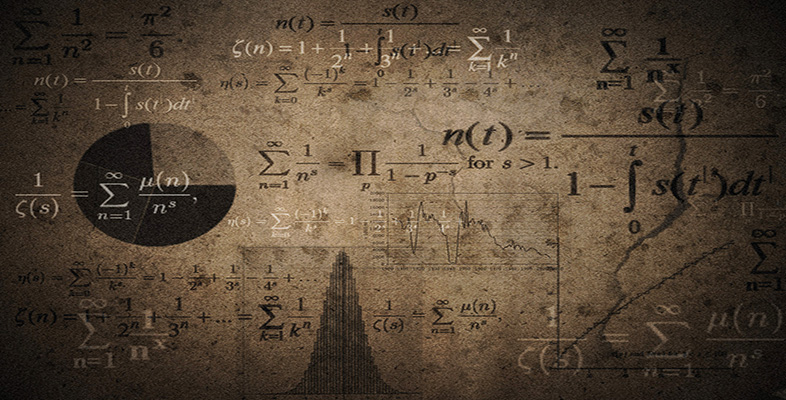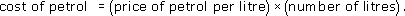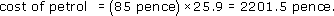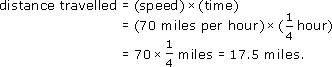Language, notation and formulas

This free course is available to start right now. Review the full course description and key learning outcomes and create an account and enrol if you want a free statement of participation.

Free course

# 3.2 Using formulas

Formulas are important because they describe general relationships, rather than specific numerical ones. For example, the tins of paint formula applies to every wall. To use such a formula you need to substitute specific values for the general terms, as the following examples show.

## Example 8

If the price of petrol is 85 pence per litre and a driver buys 25.9 litres, what is the cost?

The formula isThe price per litre is 85 pence and the number of litres is 25.9. SoRounded to the nearest penny, this is 2202 pence or £22.02.

## Example 9

Suppose you are travelling on a UK motorway in a coach and you want to know how far the coach has travelled in the previous quarter of an hour. You glance over the shoulder of the driver and you see that the speed is a steady 70 miles per hour. Estimate how far you have travelled on the motorway in the last quarter of an hour, assuming a constant speed of 70 miles per hour.

The formula givesMU120_4M6